# Mean State

Period Mean (original grids) [Watt m-2]
Model Period Mean (intersection) [Watt m-2]
Model Period Mean (complement) [Watt m-2]
Benchmark Period Mean (intersection) [Watt m-2]
Benchmark Period Mean (complement) [Watt m-2]
Bias [Watt m-2]
RMSE [Watt m-2]
Phase Shift [months]
Bias Score 
RMSE Score 
Seasonal Cycle Score 
Spatial Distribution Score 
Interannual Variability Score 
Overall Score 
Benchmark [-] 179.
CLM4 [-] 182. 182. 177. 216. 5.16 16.6 0.531 0.637 0.523 0.933 0.915 0.727 0.710
CLM4.5 [-] 183. 183. 177. 216. 5.50 16.8 0.545 0.623 0.522 0.931 0.928 0.729 0.709
CLM5 [-] 183. 183. 177. 216. 5.83 16.7 0.533 0.627 0.521 0.930 0.936 0.731 0.711
Period Mean (original grids) [Watt m-2]
Model Period Mean (intersection) [Watt m-2]
Model Period Mean (complement) [Watt m-2]
Benchmark Period Mean (intersection) [Watt m-2]
Benchmark Period Mean (complement) [Watt m-2]
Bias [Watt m-2]
RMSE [Watt m-2]
Phase Shift [months]
Bias Score 
RMSE Score 
Seasonal Cycle Score 
Spatial Distribution Score 
Interannual Variability Score 
Overall Score 
Benchmark [-] 189.
CLM4 [-] 193. 193. 188. 224. 5.16 15.8 0.379 0.697 0.536 0.971 0.999 0.785 0.754
CLM4.5 [-] 194. 194. 188. 224. 6.02 16.3 0.394 0.677 0.534 0.968 0.999 0.787 0.750
CLM5 [-] 195. 195. 188. 224. 6.89 16.6 0.458 0.662 0.533 0.955 0.998 0.783 0.744
Period Mean (original grids) [Watt m-2]
Model Period Mean (intersection) [Watt m-2]
Model Period Mean (complement) [Watt m-2]
Benchmark Period Mean (intersection) [Watt m-2]
Benchmark Period Mean (complement) [Watt m-2]
Bias [Watt m-2]
RMSE [Watt m-2]
Phase Shift [months]
Bias Score 
RMSE Score 
Seasonal Cycle Score 
Spatial Distribution Score 
Interannual Variability Score 
Overall Score 
Benchmark [-] 194.
CLM4 [-] 196. 196. 192. 221. 3.08 18.5 0.555 0.733 0.562 0.925 0.957 0.669 0.735
CLM4.5 [-] 196. 196. 192. 221. 3.59 18.8 0.575 0.720 0.560 0.923 0.956 0.671 0.732
CLM5 [-] 197. 197. 192. 221. 3.85 18.7 0.529 0.718 0.563 0.926 0.960 0.668 0.733
Period Mean (original grids) [Watt m-2]
Model Period Mean (intersection) [Watt m-2]
Model Period Mean (complement) [Watt m-2]
Benchmark Period Mean (intersection) [Watt m-2]
Benchmark Period Mean (complement) [Watt m-2]
Bias [Watt m-2]
RMSE [Watt m-2]
Phase Shift [months]
Bias Score 
RMSE Score 
Seasonal Cycle Score 
Spatial Distribution Score 
Interannual Variability Score 
Overall Score 
Benchmark [-] 81.3
CLM4 [-] 66.3 66.4 80.9 86.2 -14.7 25.3 0.338 0.805 0.717 0.978 0.944 0.768 0.822
CLM4.5 [-] 71.3 71.4 80.9 86.2 -10.5 21.0 0.213 0.853 0.749 0.986 0.893 0.707 0.823
CLM5 [-] 72.8 72.9 80.9 86.2 -9.00 20.1 0.218 0.871 0.754 0.986 0.924 0.696 0.831
Period Mean (original grids) [Watt m-2]
Model Period Mean (intersection) [Watt m-2]
Model Period Mean (complement) [Watt m-2]
Benchmark Period Mean (intersection) [Watt m-2]
Benchmark Period Mean (complement) [Watt m-2]
Bias [Watt m-2]
RMSE [Watt m-2]
Phase Shift [months]
Bias Score 
RMSE Score 
Seasonal Cycle Score 
Spatial Distribution Score 
Interannual Variability Score 
Overall Score 
Benchmark [-] 189.
CLM4 [-] 177. 177. 183. 210. -1.97 20.6 0.688 0.610 0.454 0.905 0.720 0.711 0.642
CLM4.5 [-] 178. 177. 183. 210. -1.82 20.6 0.686 0.607 0.454 0.906 0.714 0.712 0.641
CLM5 [-] 177. 177. 183. 210. -2.07 20.5 0.665 0.611 0.455 0.909 0.728 0.713 0.645
Period Mean (original grids) [Watt m-2]
Model Period Mean (intersection) [Watt m-2]
Model Period Mean (complement) [Watt m-2]
Benchmark Period Mean (intersection) [Watt m-2]
Benchmark Period Mean (complement) [Watt m-2]
Bias [Watt m-2]
RMSE [Watt m-2]
Phase Shift [months]
Bias Score 
RMSE Score 
Seasonal Cycle Score 
Spatial Distribution Score 
Interannual Variability Score 
Overall Score 
Benchmark [-] 105.
CLM4 [-] 102. 102. 104. 127. -2.21 15.7 0.0825 0.938 0.807 0.995 0.982 0.648 0.863
CLM4.5 [-] 99.6 99.6 104. 127. -4.37 17.6 0.0854 0.919 0.792 0.994 0.984 0.650 0.855
CLM5 [-] 100. 100. 104. 127. -3.81 16.7 0.0856 0.929 0.798 0.994 0.998 0.644 0.860
Period Mean (original grids) [Watt m-2]
Model Period Mean (intersection) [Watt m-2]
Model Period Mean (complement) [Watt m-2]
Benchmark Period Mean (intersection) [Watt m-2]
Benchmark Period Mean (complement) [Watt m-2]
Bias [Watt m-2]
RMSE [Watt m-2]
Phase Shift [months]
Bias Score 
RMSE Score 
Seasonal Cycle Score 
Spatial Distribution Score 
Interannual Variability Score 
Overall Score 
Benchmark [-] 140.
CLM4 [-] 135. 134. 139. 154. -4.57 17.4 0.219 0.878 0.744 0.981 0.992 0.624 0.827
CLM4.5 [-] 134. 134. 139. 154. -5.35 17.6 0.209 0.869 0.745 0.983 0.995 0.621 0.826
CLM5 [-] 136. 135. 139. 154. -3.72 16.9 0.242 0.891 0.744 0.980 0.998 0.623 0.830
Period Mean (original grids) [Watt m-2]
Model Period Mean (intersection) [Watt m-2]
Model Period Mean (complement) [Watt m-2]
Benchmark Period Mean (intersection) [Watt m-2]
Benchmark Period Mean (complement) [Watt m-2]
Bias [Watt m-2]
RMSE [Watt m-2]
Phase Shift [months]
Bias Score 
RMSE Score 
Seasonal Cycle Score 
Spatial Distribution Score 
Interannual Variability Score 
Overall Score 
Benchmark [-] 180.
CLM4 [-] 174. 174. 178. 210. -2.65 19.1 0.305 0.800 0.618 0.979 0.961 0.645 0.770
CLM4.5 [-] 174. 174. 178. 210. -2.23 19.1 0.279 0.796 0.618 0.981 0.947 0.638 0.767
CLM5 [-] 175. 175. 178. 210. -1.80 18.7 0.253 0.806 0.620 0.983 0.920 0.634 0.764
Period Mean (original grids) [Watt m-2]
Model Period Mean (intersection) [Watt m-2]
Model Period Mean (complement) [Watt m-2]
Benchmark Period Mean (intersection) [Watt m-2]
Benchmark Period Mean (complement) [Watt m-2]
Bias [Watt m-2]
RMSE [Watt m-2]
Phase Shift [months]
Bias Score 
RMSE Score 
Seasonal Cycle Score 
Spatial Distribution Score 
Interannual Variability Score 
Overall Score 
Benchmark [-] 74.6
CLM4 [-] 58.5 58.9 74.6 74.8 -16.2 22.4 0.224 0.780 0.722 0.985 0.928 0.717 0.809
CLM4.5 [-] 61.2 61.6 74.6 74.8 -13.8 20.5 0.265 0.811 0.735 0.982 0.916 0.677 0.809
CLM5 [-] 64.0 64.4 74.6 74.8 -11.2 19.0 0.311 0.844 0.742 0.979 0.929 0.675 0.819
Period Mean (original grids) [Watt m-2]
Model Period Mean (intersection) [Watt m-2]
Model Period Mean (complement) [Watt m-2]
Benchmark Period Mean (intersection) [Watt m-2]
Benchmark Period Mean (complement) [Watt m-2]
Bias [Watt m-2]
RMSE [Watt m-2]
Phase Shift [months]
Bias Score 
RMSE Score 
Seasonal Cycle Score 
Spatial Distribution Score 
Interannual Variability Score 
Overall Score 
Benchmark [-] 193.
CLM4 [-] 191. 191. 193. 202. -3.08 17.9 0.169 0.858 0.708 0.987 0.998 0.614 0.812
CLM4.5 [-] 191. 191. 193. 202. -3.11 17.9 0.154 0.859 0.708 0.989 0.997 0.615 0.813
CLM5 [-] 192. 192. 193. 202. -1.99 18.1 0.222 0.852 0.704 0.983 0.999 0.615 0.809
Period Mean (original grids) [Watt m-2]
Model Period Mean (intersection) [Watt m-2]
Model Period Mean (complement) [Watt m-2]
Benchmark Period Mean (intersection) [Watt m-2]
Benchmark Period Mean (complement) [Watt m-2]
Bias [Watt m-2]
RMSE [Watt m-2]
Phase Shift [months]
Bias Score 
RMSE Score 
Seasonal Cycle Score 
Spatial Distribution Score 
Interannual Variability Score 
Overall Score 
Benchmark [-] 120.
CLM4 [-] 112. 112. 117. 151. -2.82 17.7 0.335 0.894 0.790 0.977 0.990 0.642 0.847
CLM4.5 [-] 113. 113. 117. 151. -1.86 16.6 0.303 0.905 0.798 0.979 0.998 0.629 0.851
CLM5 [-] 113. 113. 117. 151. -1.95 16.4 0.358 0.908 0.800 0.975 0.998 0.623 0.851
Period Mean (original grids) [Watt m-2]
Model Period Mean (intersection) [Watt m-2]
Model Period Mean (complement) [Watt m-2]
Benchmark Period Mean (intersection) [Watt m-2]
Benchmark Period Mean (complement) [Watt m-2]
Bias [Watt m-2]
RMSE [Watt m-2]
Phase Shift [months]
Bias Score 
RMSE Score 
Seasonal Cycle Score 
Spatial Distribution Score 
Interannual Variability Score 
Overall Score 
Benchmark [-] 168.
CLM4 [-] 135. 135. 143. 180. -6.91 19.6 0.271 0.779 0.669 0.973 0.965 0.631 0.781
CLM4.5 [-] 136. 136. 143. 180. -6.33 19.0 0.272 0.785 0.672 0.973 0.972 0.629 0.784
CLM5 [-] 137. 137. 143. 180. -5.85 18.9 0.278 0.788 0.672 0.972 0.967 0.624 0.783
Period Mean (original grids) [Watt m-2]
Model Period Mean (intersection) [Watt m-2]
Model Period Mean (complement) [Watt m-2]
Benchmark Period Mean (intersection) [Watt m-2]
Benchmark Period Mean (complement) [Watt m-2]
Bias [Watt m-2]
RMSE [Watt m-2]
Phase Shift [months]
Bias Score 
RMSE Score 
Seasonal Cycle Score 
Spatial Distribution Score 
Interannual Variability Score 
Overall Score 
Benchmark [-] 175.
CLM4 [-] 160. 160. 173. 224. -12.1 20.3 0.278 0.701 0.733 0.977 0.989 0.574 0.785
CLM4.5 [-] 161. 161. 173. 224. -11.6 20.0 0.273 0.705 0.734 0.977 0.989 0.573 0.785
CLM5 [-] 162. 162. 173. 224. -10.2 19.7 0.305 0.716 0.728 0.974 0.988 0.578 0.785
Period Mean (original grids) [Watt m-2]
Model Period Mean (intersection) [Watt m-2]
Model Period Mean (complement) [Watt m-2]
Benchmark Period Mean (intersection) [Watt m-2]
Benchmark Period Mean (complement) [Watt m-2]
Bias [Watt m-2]
RMSE [Watt m-2]
Phase Shift [months]
Bias Score 
RMSE Score 
Seasonal Cycle Score 
Spatial Distribution Score 
Interannual Variability Score 
Overall Score 
Benchmark [-] 80.0
CLM4 [-] 67.4 67.3 79.8 82.9 -13.6 22.1 0.118 0.812 0.746 0.992 0.903 0.772 0.829
CLM4.5 [-] 70.5 70.3 79.8 82.9 -11.0 19.4 0.109 0.839 0.764 0.993 0.904 0.731 0.832
CLM5 [-] 70.5 70.4 79.8 82.9 -10.9 19.6 0.0911 0.839 0.762 0.994 0.890 0.724 0.829
Period Mean (original grids) [Watt m-2]
Model Period Mean (intersection) [Watt m-2]
Model Period Mean (complement) [Watt m-2]
Benchmark Period Mean (intersection) [Watt m-2]
Benchmark Period Mean (complement) [Watt m-2]
Bias [Watt m-2]
RMSE [Watt m-2]
Phase Shift [months]
Bias Score 
RMSE Score 
Seasonal Cycle Score 
Spatial Distribution Score 
Interannual Variability Score 
Overall Score 
Benchmark [-] 205.
CLM4 [-] 192. 192. 200. 227. -6.15 20.3 0.271 0.759 0.637 0.970 0.901 0.625 0.755
CLM4.5 [-] 191. 192. 200. 227. -6.61 20.3 0.298 0.766 0.636 0.967 0.898 0.627 0.755
CLM5 [-] 192. 192. 200. 227. -6.23 20.2 0.250 0.768 0.638 0.975 0.895 0.627 0.757
Period Mean (original grids) [Watt m-2]
Model Period Mean (intersection) [Watt m-2]
Model Period Mean (complement) [Watt m-2]
Benchmark Period Mean (intersection) [Watt m-2]
Benchmark Period Mean (complement) [Watt m-2]
Bias [Watt m-2]
RMSE [Watt m-2]
Phase Shift [months]
Bias Score 
RMSE Score 
Seasonal Cycle Score 
Spatial Distribution Score 
Interannual Variability Score 
Overall Score 
Benchmark [-] 153.
CLM4 [-] 146. 146. 151. 194. -3.56 15.8 0.146 0.929 0.769 0.990 0.999 0.621 0.846
CLM4.5 [-] 145. 145. 151. 194. -4.37 15.8 0.143 0.917 0.770 0.990 1.00 0.622 0.845
CLM5 [-] 144. 144. 151. 194. -5.31 16.1 0.154 0.907 0.770 0.990 1.00 0.618 0.842
Period Mean (original grids) [Watt m-2]
Model Period Mean (intersection) [Watt m-2]
Model Period Mean (complement) [Watt m-2]
Benchmark Period Mean (intersection) [Watt m-2]
Benchmark Period Mean (complement) [Watt m-2]
Bias [Watt m-2]
RMSE [Watt m-2]
Phase Shift [months]
Bias Score 
RMSE Score 
Seasonal Cycle Score 
Spatial Distribution Score 
Interannual Variability Score 
Overall Score 
Benchmark [-] 115.
CLM4 [-] 111. 111. 114. 123. -3.01 15.2 0.0925 0.918 0.823 0.994 0.981 0.651 0.865
CLM4.5 [-] 112. 112. 114. 123. -2.43 14.6 0.0955 0.925 0.826 0.994 0.996 0.628 0.866
CLM5 [-] 111. 112. 114. 123. -2.98 14.7 0.0893 0.924 0.826 0.994 0.999 0.636 0.867
Period Mean (original grids) [Watt m-2]
Model Period Mean (intersection) [Watt m-2]
Model Period Mean (complement) [Watt m-2]
Benchmark Period Mean (intersection) [Watt m-2]
Benchmark Period Mean (complement) [Watt m-2]
Bias [Watt m-2]
RMSE [Watt m-2]
Phase Shift [months]
Bias Score 
RMSE Score 
Seasonal Cycle Score 
Spatial Distribution Score 
Interannual Variability Score 
Overall Score 
Benchmark [-] 162.
CLM4 [-] 157. 157. 162. 171. -4.35 15.9 0.143 0.916 0.805 0.991 0.999 0.648 0.861
CLM4.5 [-] 156. 156. 162. 171. -5.47 15.9 0.139 0.903 0.810 0.991 1.00 0.655 0.862
CLM5 [-] 159. 159. 162. 171. -2.78 15.1 0.159 0.930 0.810 0.989 1.00 0.648 0.865
Period Mean (original grids) [Watt m-2]
Model Period Mean (intersection) [Watt m-2]
Model Period Mean (complement) [Watt m-2]
Benchmark Period Mean (intersection) [Watt m-2]
Benchmark Period Mean (complement) [Watt m-2]
Bias [Watt m-2]
RMSE [Watt m-2]
Phase Shift [months]
Bias Score 
RMSE Score 
Seasonal Cycle Score 
Spatial Distribution Score 
Interannual Variability Score 
Overall Score 
Benchmark [-] 176.
CLM4 [-] 174. 174. 174. 226. 0.0995 17.1 0.485 0.825 0.468 0.939 0.951 0.645 0.716
CLM4.5 [-] 174. 174. 174. 226. 0.344 17.2 0.512 0.828 0.467 0.935 0.945 0.645 0.714
CLM5 [-] 174. 174. 174. 226. 0.173 17.1 0.479 0.828 0.468 0.940 0.943 0.645 0.715
Period Mean (original grids) [Watt m-2]
Model Period Mean (intersection) [Watt m-2]
Model Period Mean (complement) [Watt m-2]
Benchmark Period Mean (intersection) [Watt m-2]
Benchmark Period Mean (complement) [Watt m-2]
Bias [Watt m-2]
RMSE [Watt m-2]
Phase Shift [months]
Bias Score 
RMSE Score 
Seasonal Cycle Score 
Spatial Distribution Score 
Interannual Variability Score 
Overall Score 
Benchmark [-] 182.
CLM4 [-] 181. 181. 181. 201. -0.244 19.2 0.361 0.883 0.570 0.957 0.996 0.616 0.765
CLM4.5 [-] 181. 181. 181. 201. -0.363 19.2 0.395 0.888 0.569 0.950 0.995 0.616 0.765
CLM5 [-] 180. 181. 181. 201. -0.876 19.1 0.319 0.886 0.572 0.959 0.999 0.614 0.767
Period Mean (original grids) [Watt m-2]
Model Period Mean (intersection) [Watt m-2]
Model Period Mean (complement) [Watt m-2]
Benchmark Period Mean (intersection) [Watt m-2]
Benchmark Period Mean (complement) [Watt m-2]
Bias [Watt m-2]
RMSE [Watt m-2]
Phase Shift [months]
Bias Score 
RMSE Score 
Seasonal Cycle Score 
Spatial Distribution Score 
Interannual Variability Score 
Overall Score 
Benchmark [-] 176.
CLM4 [-] 174. 173. 176. 178. -5.68 21.2 0.306 0.837 0.697 0.970 0.998 0.607 0.801
CLM4.5 [-] 175. 174. 176. 178. -5.09 20.9 0.287 0.841 0.698 0.972 0.999 0.604 0.802
CLM5 [-] 176. 175. 176. 178. -3.76 20.8 0.301 0.842 0.697 0.970 0.999 0.603 0.801

# Temporally integrated period mean

BENCHMARK MEAN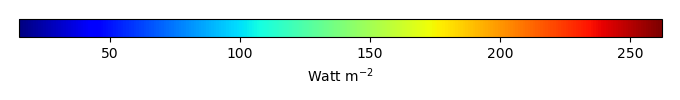MODEL MEANBIAS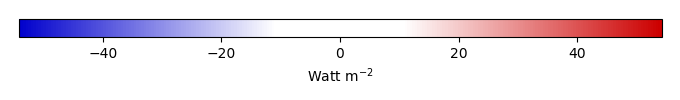BIAS SCORERMSE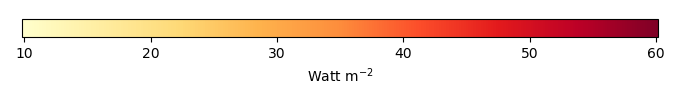RMSE SCOREBENCHMARK INTERANNUAL VARIABILITY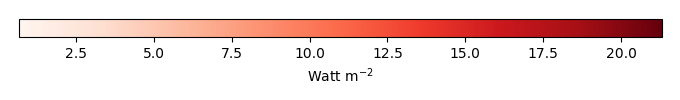MODEL INTERANNUAL VARIABILITYINTERANNUAL VARIABILITY SCOREBENCHMARK MAX MONTHMODEL MAX MONTHDIFFERENCE IN MAX MONTH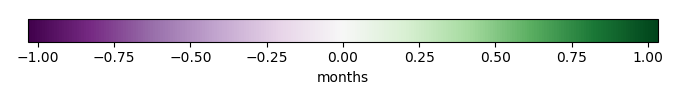SEASONAL CYCLE SCORESPATIAL TAYLOR DIAGRAMMODEL COLORS# Spatially integrated regional mean

MODEL COLORSREGIONAL MEANANNUAL CYCLEMONTHLY ANOMALYANNUAL CYCLE# All Models

BenchmarkCLM4CLM4.5CLM5# Data Information

creation_date: Tue Jul 1 08:29:23 PDT 2014

source_file: This product is generated from monthly 1 degree GEWEX SRB Radiation observations